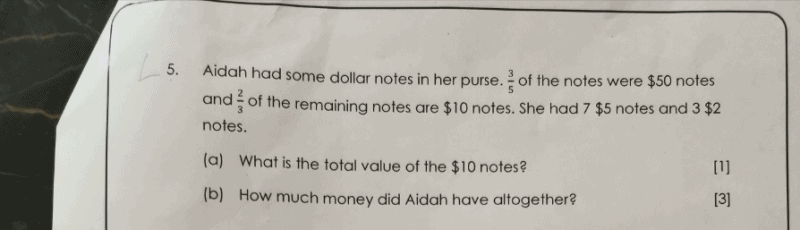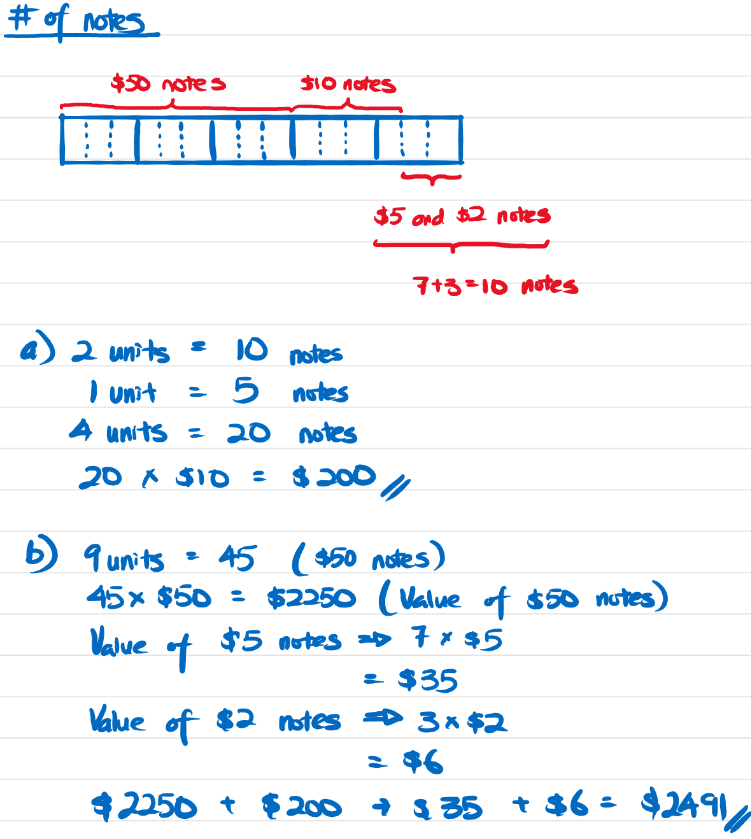# QuestionSource: Assessment

Draw a model with 15 parts.

3/5 of the notes are \$50, hence it has 9 parts.

2/3 of the remaining notes are \$10 notes (15-9=6, the remaining notes have 6 parts).

Hence 2/3 x 6 = 4 parts. \$10 notes has 4 parts

There are 7 \$5 notes and 3 \$2 notes that take up the last 2 parts.

2 parts = 10 notes

1 part = 5 notes.

No of \$10 notes = 5×4 =20

Total value of \$10 notes = 20x\$10 = \$200 (ans)

No of \$50 notes = 5×9=45

Total value of \$50 notes = 45×50 = \$2250

Total value of all notes = \$2250 + \$200 + 7×5 + 3×2 =\$2491 (ans)

0 Replies 1 Like0 Replies 2 Likes